SSC CGL Previous Year Questions: Geometry- 4

# SSC CGL Previous Year Questions: Geometry- 4 - SSC CGL

Test Description

## 40 Questions MCQ Test SSC CGL (Tier - 1) - Previous Year Papers (Topic Wise) - SSC CGL Previous Year Questions: Geometry- 4

SSC CGL Previous Year Questions: Geometry- 4 for SSC CGL 2023 is part of SSC CGL (Tier - 1) - Previous Year Papers (Topic Wise) preparation. The SSC CGL Previous Year Questions: Geometry- 4 questions and answers have been prepared according to the SSC CGL exam syllabus.The SSC CGL Previous Year Questions: Geometry- 4 MCQs are made for SSC CGL 2023 Exam. Find important definitions, questions, notes, meanings, examples, exercises, MCQs and online tests for SSC CGL Previous Year Questions: Geometry- 4 below.
Solutions of SSC CGL Previous Year Questions: Geometry- 4 questions in English are available as part of our SSC CGL (Tier - 1) - Previous Year Papers (Topic Wise) for SSC CGL & SSC CGL Previous Year Questions: Geometry- 4 solutions in Hindi for SSC CGL (Tier - 1) - Previous Year Papers (Topic Wise) course. Download more important topics, notes, lectures and mock test series for SSC CGL Exam by signing up for free. Attempt SSC CGL Previous Year Questions: Geometry- 4 | 40 questions in 40 minutes | Mock test for SSC CGL preparation | Free important questions MCQ to study SSC CGL (Tier - 1) - Previous Year Papers (Topic Wise) for SSC CGL Exam | Download free PDF with solutions
 1 Crore+ students have signed up on EduRev. Have you?
SSC CGL Previous Year Questions: Geometry- 4 - Question 1

### If the sides of a right angled triangle are three consecutive integers, then the length of the smallest side is    (SSC CHSL 2014)

Detailed Solution for SSC CGL Previous Year Questions: Geometry- 4 - Question 1

Let the three sides are x, (x + 1) and (x + 2)
In a  right angle triangle, sum of square of base and
height is equal to the square of hypotenuse
∴ (x + 2)2 = x2 + (x + 1)2
4x + 4 = x2 + 2x + 1
x2 – 2x – 3 = 0
(x – 3) (x + 1) = 0 ⇒ x  = 3
Thus, three sides are x = 3 unit
x + 1 = 3 + 1 = 4 unit
x + 2 = 3 + 2 = 5 unit
Smallest side = x = 3 unit.

SSC CGL Previous Year Questions: Geometry- 4 - Question 2

### The perimeters of two similar triangles ΔABC and ΔPQR are 36 cm and 24 cm respectively. If PQ = 10 cm, the AB is    (SSC CHSL 2014)

Detailed Solution for SSC CGL Previous Year Questions: Geometry- 4 - Question 2

ΔABC ~ ΔPQR (given)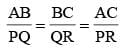(Corresponding sides are proportional)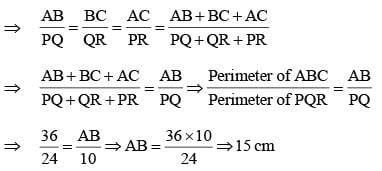SSC CGL Previous Year Questions: Geometry- 4 - Question 3

### In ΔABC, D and E are two points on the sides AB and AC respectively so that DE || BC and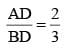. Then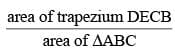is equal to    (SSC CHSL 2014)

Detailed Solution for SSC CGL Previous Year Questions: Geometry- 4 - Question 3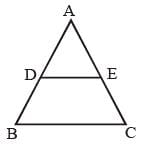Since DE is parallel to BC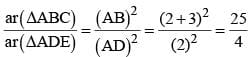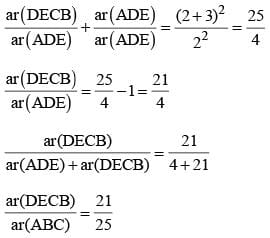SSC CGL Previous Year Questions: Geometry- 4 - Question 4

The sum of the interior angles of a polygon is 1444°. The number of sides of the polygon is    (SSC CHSL 2014)

Detailed Solution for SSC CGL Previous Year Questions: Geometry- 4 - Question 4

Sum of interior angles of polygon = (n – 2) × 180°
(n – 2) × 180° = 1440
n – 2 = 1440/180 = 8
n = 10
Hence, the number of sides is 10.

SSC CGL Previous Year Questions: Geometry- 4 - Question 5

If AB = 5 cm, AC = 12 and AB ⊥ AC then the radius of the circumcircle of ΔABC is   (SSC Sub. Ins. 2014)

Detailed Solution for SSC CGL Previous Year Questions: Geometry- 4 - Question 5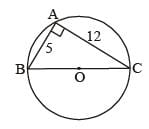In ΔABC, AB ⊥ AC,
BC2 = AB2 + AC2
BC2 = (5)2 + (12)2
BC2 = 25 + 144
BC2 = 169
BC = √169 = 13 cm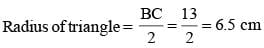SSC CGL Previous Year Questions: Geometry- 4 - Question 6

Two circles with radii 25 cm and 9 cm touch each other externally. The length of the direct common tangent is   (SSC Sub. Ins. 2014)

Detailed Solution for SSC CGL Previous Year Questions: Geometry- 4 - Question 6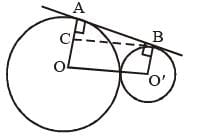Let two circles with center O and O' and radius
OA = 25 cm and O' B = 9 cm.
draw BC || OO'
then O' B = OC = 9 cm
AC = 25 – 9 = 16 cm
From ΔABC,
BC = OO' = 25 + 9 = 34 cm
AC = 16 cm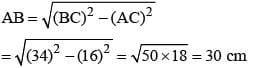SSC CGL Previous Year Questions: Geometry- 4 - Question 7

In a ΔABC,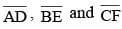are three medians. Then the ratio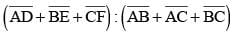is    (SSC Sub. Ins. 2014)

Detailed Solution for SSC CGL Previous Year Questions: Geometry- 4 - Question 7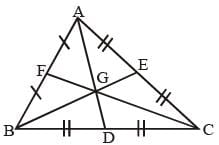In a triangle sum of two sides are always greater than third sides and point of intersection of medians of a triangle is called centroid of that triangle, which divides the median into 2 : 1 ratio.
∴ BG : GE = 2 : 1; CG : GF = 2 : 1
AG : GD = 2 : 1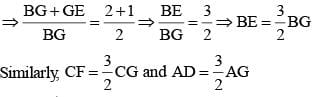Now, In ΔBGC, BG + GC > BC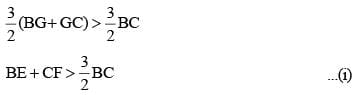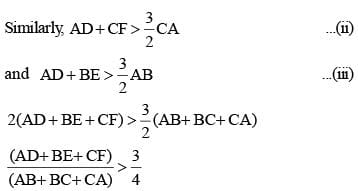SSC CGL Previous Year Questions: Geometry- 4 - Question 8

In a ΔABC, AD, BE and CF are three medians. The perimeter of ΔABC is always   (SSC Sub. Ins. 2014)

Detailed Solution for SSC CGL Previous Year Questions: Geometry- 4 - Question 8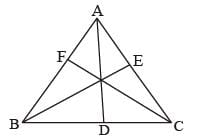Let ABC be the triangle and D, E and F are midpoints of BC, CA and AB respectively.
AB + AC > 2 AD
Similarly, we get
BC + AC > 2 CF
BC + AB > 2 BE
On adding the above inequations, we get
(AB + AC + BC + AC + BC + AB) > 2(AD + BE + CF)
2 (AB + AC + BC) > 2 (AD + BE + CF)
∴ AB + BC + BC > AD + BE + CF
Thus, the perimeter of triangle is greater than the sum of the medians.

SSC CGL Previous Year Questions: Geometry- 4 - Question 9

In a ΔABC,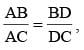∠B = 70° and ∠C = 50°, then ∠BAD =?   (SSC Sub. Ins. 2014)

Detailed Solution for SSC CGL Previous Year Questions: Geometry- 4 - Question 9

In ΔABC, If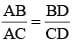then by the converse of internal angle bisector theorem, we get that AD bisects angle A.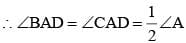Again, we know that, sum of angles in a triangle = 180°
∴ ∠A + ∠B + ∠C = 180°
∠A + 70° + 50° = 180° ⇒ ∠A = 60°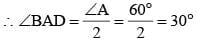SSC CGL Previous Year Questions: Geometry- 4 - Question 10

A, B and C are the three points on a circle such that the angles subtended by the chords AB and AC at the centre O are 90° and 110° respectively. ∠BAC is equal to    (SSC CGL 1st Sit. 2013)

Detailed Solution for SSC CGL Previous Year Questions: Geometry- 4 - Question 10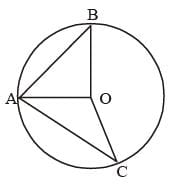∠AOB = 90° ; OA = OB = r
∴ ∠BAO = ∠ABO = 45°
∴ ∠AOC = 110°; OA = OC = r
∴ ∠OAC = ∠OCA = 70/2 = 35°
∴ ∠BAC = 45° + 35° = 80°

SSC CGL Previous Year Questions: Geometry- 4 - Question 11

In ΔABC, DE || AC. D and E are two points on AB and CB respectively. If AB = 10 cm and AD 2.4 cm, then BE : CE is   (SSC CGL 1st Sit. 2013)

Detailed Solution for SSC CGL Previous Year Questions: Geometry- 4 - Question 11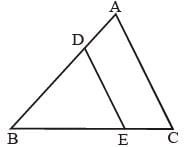From question, DE  || AC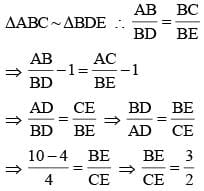SSC CGL Previous Year Questions: Geometry- 4 - Question 12

Two parallel chords of a circle, of diameter 20 cm lying on the opposite sides of the centre are of lengths 12 cm and 16 cm.
The distance between the chords is    (SSC CGL 1st Sit. 2013)

Detailed Solution for SSC CGL Previous Year Questions: Geometry- 4 - Question 12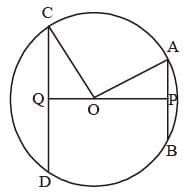OA = OC = 10 cm (radius)
AB = 12 cm
AP = PB = 6 cm
CD = 16 cm
CQ = QD = 8 cm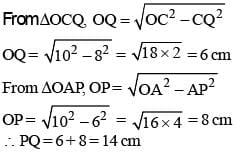SSC CGL Previous Year Questions: Geometry- 4 - Question 13

The height of an equilateral triangle is 15 cm. The area of the triangle is    (SSC CGL 1st Sit. 2013)

Detailed Solution for SSC CGL Previous Year Questions: Geometry- 4 - Question 13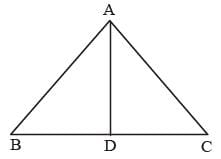Let, AB = BC = CA = 2a cm,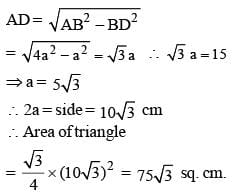SSC CGL Previous Year Questions: Geometry- 4 - Question 14

A cyclic quadrilateral ABCD is such that AB = BC, AD = DC, AC ⊥ BD, ∠CAD = θ. Then the angle ∠ABC =   (SSC CGL 1st Sit. 2013)

Detailed Solution for SSC CGL Previous Year Questions: Geometry- 4 - Question 14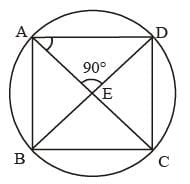∠B + ∠D = 180°
∠A + ∠C = 180°
∠DAC = θ {given}
∠AED = 90° {given}
In ΔAED,
∴ ∠ADE = 90° – θ = ∠CDE
∴ ∠ABC = 180° – 2 (90° – θ) = 2θ

SSC CGL Previous Year Questions: Geometry- 4 - Question 15

The length of the tangent drawn to a circle of radius 4 cm from a point 5 cm away from the centre of the circle is   (SSC CGL 1st Sit. 2013)

Detailed Solution for SSC CGL Previous Year Questions: Geometry- 4 - Question 15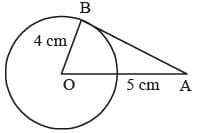Here OB = 4 cm (radius)
OA = 5 cm
As AB is a tangent to the circle,
∴ ∠OBA = 90°
OA = 5, OB = 4SSC CGL Previous Year Questions: Geometry- 4 - Question 16

In ΔABC, ∠A + ∠B = 65°, ∠B + ∠C = 140°, then find ∠B.   (SSC CGL 1st Sit. 2013)

Detailed Solution for SSC CGL Previous Year Questions: Geometry- 4 - Question 16

∠A + ∠B = 65°
∴ ∠C = 180° – 65° = 115°
∠B + ∠C = 140°
∴ ∠B = 140° – 115° = 25°

SSC CGL Previous Year Questions: Geometry- 4 - Question 17

The sum of three altitudes of a triangle is   (SSC CGL 1st Sit. 2013)

Detailed Solution for SSC CGL Previous Year Questions: Geometry- 4 - Question 17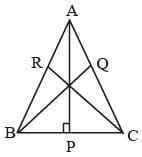We know that Altitude from a point on a line is the shortest distance of that point from the line.
AP <  AB
BQ < BC
CR <  AC
∴ AP + BQ + CR < AB + BC + AC

SSC CGL Previous Year Questions: Geometry- 4 - Question 18

If the inradius of a triangle with perimeter 32 cm is 6 cm, then the area of the triangle (in sq. cm) is   (SSC CGL 1st Sit. 2013)

Detailed Solution for SSC CGL Previous Year Questions: Geometry- 4 - Question 18

Area of triangle = Inradius × Semi-perimeter
= 6 ×16 = 96  sq. cm.

SSC CGL Previous Year Questions: Geometry- 4 - Question 19

D and E are the mid-points of AB and AC of ΔABC. If ∠A = 80°, ∠C = 35°, then ∠EDB is equal to   (SSC CGL 1st Sit. 2013)

Detailed Solution for SSC CGL Previous Year Questions: Geometry- 4 - Question 19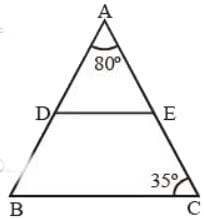As D and E are mid point so,
DE is parallel to BC
So ∠AED = ∠C = 35°
Since ∠A= 80°
So, ∠EDB = 180° - ∠ADE
= 180° - 65° =115°

SSC CGL Previous Year Questions: Geometry- 4 - Question 20

When two circles touch externally, the number of common tangents are    (SSC CGL 1st Sit. 2013)

Detailed Solution for SSC CGL Previous Year Questions: Geometry- 4 - Question 20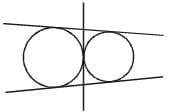Number of common tengent = 3

SSC CGL Previous Year Questions: Geometry- 4 - Question 21

Two circles of equal radii touch externally at a point P. From a point T on the tangent at P, tangents TQ and TR are drawn to the circles with points of contact Q and R respectively. The relation of TQ and TR is    (SSC CGL 1st Sit. 2013)

Detailed Solution for SSC CGL Previous Year Questions: Geometry- 4 - Question 21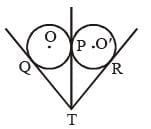Let two circles with centre O and O' touches each other at point P. From point T tangent TQ and TR are drawn on two circles equal radius.
As we know two tangent drawn from a external points are always equal
So, TQ = TP ...(i)
and TP = TR ...(ii)
from (i) and (ii), TQ = TP = TR

SSC CGL Previous Year Questions: Geometry- 4 - Question 22

ABCD is a cyclic quadrilateral, AB is a diameter of the circle. If ∠ACD = 50°, the value of ∠BAD is    (SSC CGL 1st Sit. 2013)

Detailed Solution for SSC CGL Previous Year Questions: Geometry- 4 - Question 22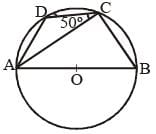In ΔABC, ∠ACB = 90°
∴ ∠ACB + ∠ACD =
90°+ 50° = 140°
As angle mode by triangle
in semicircle is equal to 90°
∴ In quad. ABCD, ∠BAD + ∠BCD = 180° (angle of opp. pair of quad is equal to 180°)
∠BAD =180° - 140° = 40°

SSC CGL Previous Year Questions: Geometry- 4 - Question 23

The length of tangent (upto the point of contact) drawn from an external point P to a circle of radius 5 cm is 12 cm. The distance of P from the centre of the circle is   (SSC CGL Ist. Sitt. 2013)

Detailed Solution for SSC CGL Previous Year Questions: Geometry- 4 - Question 23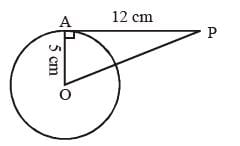AP is a tangent and OA is a radius.
Therefore, OA is ⊥ at AP.
So, In ΔOAP
OP2 = OA2 + AP2 = 52 + 122
OP2 = 25 + 144 = 169
OP = 13 cm.

SSC CGL Previous Year Questions: Geometry- 4 - Question 24

Side BC of ΔABC is produced to D. If ∠ACD = 140° and ∠ABC = 3∠BAC, then find ∠A.   (SSC CGL 1st Sit. 2013)

Detailed Solution for SSC CGL Previous Year Questions: Geometry- 4 - Question 24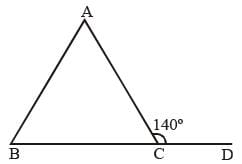∴ ∠ACB = 180º – 140º = 40º
In  ΔABC, ∠BAC + ∠ABC + ∠ACB = 180º
∠BAC + 3 ∠BAC + 40º = 180º
4∠BAC = 180º – 40º
∠BAC = 140/4 = 35º

SSC CGL Previous Year Questions: Geometry- 4 - Question 25

A, B, P are three points on a circle having centre O. If ∠OAP = 25° and ∠OBP = 35°, then the measure of ∠AOB is   (SSC CGL 1st Sit. 2013)

Detailed Solution for SSC CGL Previous Year Questions: Geometry- 4 - Question 25

In ΔOBP.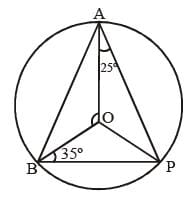∴ ∠OBP = ∠OPB = 35º
In ΔAOP
∴  ∠OAP = ∠OPA = 25º
Now, ∠APB = ∠OPA + ∠OPB = 25º + 35º = 60º
Hence, ∠AOB = 2∠APB
(Since, Angle be substended by arc at centre is twice the angle subtend at the perimeter)
= 2 × 60º = 120º

SSC CGL Previous Year Questions: Geometry- 4 - Question 26

In a triangle, if three altitudes are equal, then the triangle is   (SSC CGL 1st Sit. 2013)

Detailed Solution for SSC CGL Previous Year Questions: Geometry- 4 - Question 26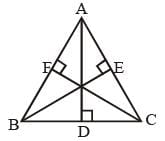Let ΔABC is a equilateral triangle of side AB = BC = AC = 2a unit.
AD, BE and CF are three altitudes in the DABC,
In equilateral triangle ∠A = ∠B = ∠C = 60°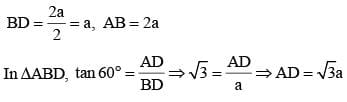Similarly, In ΔACF,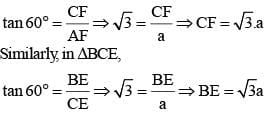Here, we get AD = BE = CF
Hence, three altitude are equal
Thus, triangle must be a equilateral triangle.

SSC CGL Previous Year Questions: Geometry- 4 - Question 27

A, B, C, D are four points on a circle. AC and BD intersect at a point E such that ∠BEC = 130° and ∠ECD = 20°. ∠BAC is   (SSC CGL 1st Sit. 2013)

Detailed Solution for SSC CGL Previous Year Questions: Geometry- 4 - Question 27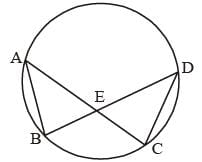∴ ∠BEC = 130°
∴ ∠DEC = 180° – 130° = 50°
∴ ∠EDC = 180° – 50° – 20° = 110°
∴ ∠BAC = ∠EDC = 110°
(Angles on the same arc)

SSC CGL Previous Year Questions: Geometry- 4 - Question 28

A vertical stick 12 cm long casts a shadow 8 cm long on the ground. At the same time, a tower casts a shadow 40 m long on the ground. The height of the tower is   (SSC CGL 1st Sit. 2013)

Detailed Solution for SSC CGL Previous Year Questions: Geometry- 4 - Question 28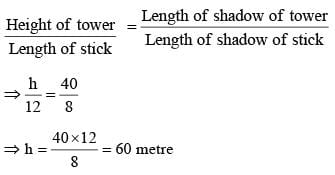SSC CGL Previous Year Questions: Geometry- 4 - Question 29

If O be the circumcentre of a triangle PQR and ∠QOR = 110°, ∠OPR = 25°, then the measure of ∠PRQ is   (SSC CGL 1st Sit. 2013)

Detailed Solution for SSC CGL Previous Year Questions: Geometry- 4 - Question 29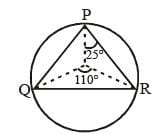In ΔOPR, OP = OR = radius
∠OPR = ∠ORP = 25°
In ΔOQR OQ = OR = radius
∠OQR= ∠ORQ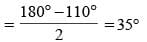∴ ∠PRQ = ∠PRO + ∠ORQ = 25° + 35° = 60°

SSC CGL Previous Year Questions: Geometry- 4 - Question 30

The diagonals AC and BD of a cyclic quadrilateral ABCD intersect each other at the point P. Then, it is always true that   (SSC CGL 1st Sit. 2013)

Detailed Solution for SSC CGL Previous Year Questions: Geometry- 4 - Question 30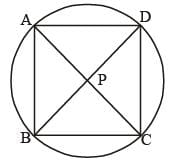Here, AC and BD are chords of the circle.
∴ AP . CP = BP . DP

SSC CGL Previous Year Questions: Geometry- 4 - Question 31

If G is the centroid of ΔABC and area of ΔABC = 48cm2, then the area of ΔBGC is   (SSC CGL 1st Sit. 2013)

Detailed Solution for SSC CGL Previous Year Questions: Geometry- 4 - Question 31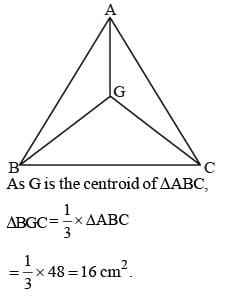SSC CGL Previous Year Questions: Geometry- 4 - Question 32

In a triangle ABC, ∠A = 90°, ∠C = 55°, AD ⊥ BC . What is the value of ∠BAD?   (SSC CGL 1st Sit. 2013)

Detailed Solution for SSC CGL Previous Year Questions: Geometry- 4 - Question 32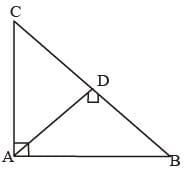∠A = 90°, ∠C = 55°
∴ ∠B = 90° – 55° = 35°
∴ ∠BAD = 90° – 35° = 55°

SSC CGL Previous Year Questions: Geometry- 4 - Question 33

N is the foot of the perpendicular from a point P of a circle with radius 7 cm, on a diameter AB of the circle. If the length of the chord PB is 12 cm, the distance of the point N from the point B is   (SSC CGL 1st Sit. 2013)

Detailed Solution for SSC CGL Previous Year Questions: Geometry- 4 - Question 33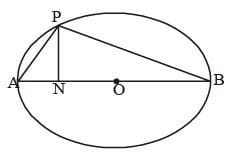AB = 14 cm, PB = 12 cm
∠APB = 90°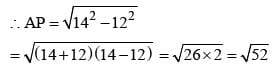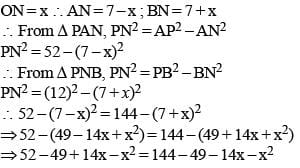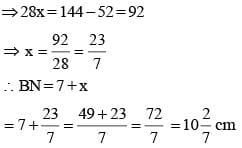SSC CGL Previous Year Questions: Geometry- 4 - Question 34

If the arcs of square length in two circles subtend angles of 60° and 75° at their centres, the ratio of their radii is (SSC CGL 2nd Sit.  2013)

Detailed Solution for SSC CGL Previous Year Questions: Geometry- 4 - Question 34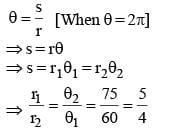SSC CGL Previous Year Questions: Geometry- 4 - Question 35

From a point P which is at a distance of 13 cm from center O of a circle of radius 5 cm, in the same plane, a pair of tangents PQ and PR are drawn to the circle. Area of quadrilateral PQOR is    (SSC CGL 2nd Sit.  2013)

Detailed Solution for SSC CGL Previous Year Questions: Geometry- 4 - Question 35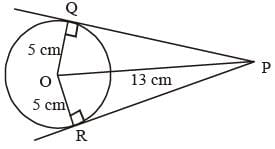In ΔOQP and ΔORP,
∠OQP = ∠ORP = 90°
PQ = PR {tangent}
∴ ΔOQP ≌ ΔORP
Area of ΔOQP = Area of ΔORP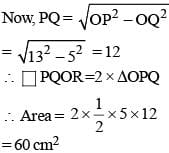SSC CGL Previous Year Questions: Geometry- 4 - Question 36

ABCD is a cyclic quadrilateral AB and DC are produced to meet at P. If ∠ADC = 70° and ∠DAB = 60°, then the ∠PBC + ∠PCB is   (SSC CGL 2nd Sit.  2013)

Detailed Solution for SSC CGL Previous Year Questions: Geometry- 4 - Question 36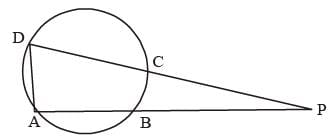As ABCD is a cyclic quadrilateral.
In which
∠ABC = 180° – 70° = 110°
⇒ ∠PBC = 180° – 110° = 70°
And ∠DAB = 60°
∠BCD = 180° – 60° = 120°
⇒ ∠PCB = 180° – 120° = 60°
∴ ∠PBC + ∠PCB = 70° + 60° = 130°

SSC CGL Previous Year Questions: Geometry- 4 - Question 37

Two circles touch each other internally. Their radii are 2 cm and 3 cm. The biggest chord of the greater circle which is outside the inner circle of length.   (SSC CGL 2nd Sit.  2013)

Detailed Solution for SSC CGL Previous Year Questions: Geometry- 4 - Question 37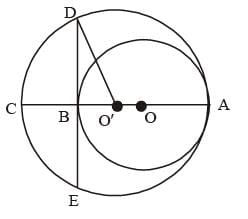O'A = 3 cm
OA = 2 cm
CA = 6 cm
O'D = 3 cm
O'B = 1 cm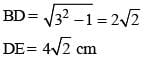SSC CGL Previous Year Questions: Geometry- 4 - Question 38

In a triangle ABC, the side BC is extended up to D. Such that CD = AC, if ∠BAD = 109° and ∠ACB = 72° then the value of ∠ABC is   (SSC CGL 2nd Sit.  2013)

Detailed Solution for SSC CGL Previous Year Questions: Geometry- 4 - Question 38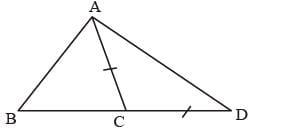∠ACD = 180° – ∠ACB = 180° – 72° = 108°
Now from ΔABD
∴ ∠ABD = 180° – 109° – 36° = 35°

SSC CGL Previous Year Questions: Geometry- 4 - Question 39

ABC is a right angled triangle, B being the right angle. Midpoints of BC and AC are respectively B' and A'. The ratio of the area of the quadrilateral AA' B'B to the area of the triangle ABC is  (SSC CGL 2nd Sit.  2013)

Detailed Solution for SSC CGL Previous Year Questions: Geometry- 4 - Question 39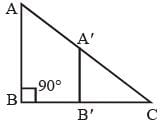B' and A' are mid-point of the side BC and AC.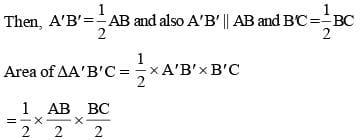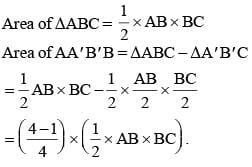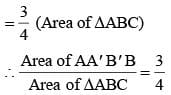SSC CGL Previous Year Questions: Geometry- 4 - Question 40

In a circle of radius 17 cm, two parallel chords of lengths 30 cm and 16 cm are drawn. If both the chords are on the same side of the centre, then the distance between the chords is   (SSC CGL 2nd Sit.  2013)

Detailed Solution for SSC CGL Previous Year Questions: Geometry- 4 - Question 40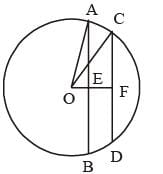AE = 15  cm
OA = 17 cm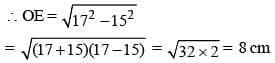CF = 8 cm
OC = 17 cm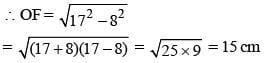Distance between two chords = OF – OE = 15 –8 = 7 cm

## SSC CGL (Tier - 1) - Previous Year Papers (Topic Wise)

250 tests
Information about SSC CGL Previous Year Questions: Geometry- 4 Page
In this test you can find the Exam questions for SSC CGL Previous Year Questions: Geometry- 4 solved & explained in the simplest way possible. Besides giving Questions and answers for SSC CGL Previous Year Questions: Geometry- 4, EduRev gives you an ample number of Online tests for practice

250 tests